Warning

SDK preview 2.10 will be released by June 6th!

# Hand-Eye Calibration Problem

This tutorial aims to describe the problem that the hand-eye calibration solves as well as to introduce robot poses and coordinate systems that are required for the hand-eye calibration. The problem is the same for eye-to-hand systems and eye-in-hand systems. Therefore, we first provide a detailed description for the eye-to-hand configuration. Then, we point out the differences for the eye-in-hand configuration. If you are not familiar with (robot) poses and coordinate systems, check out Position, Orientation and Coordinate Transformations.

## Eye-to-hand

How can a robot pick an object?

 Let’s start with a robot that doesn’t involve a camera. Its two main coordinate systems are: The robot base coordinate system The end-effector coordinate system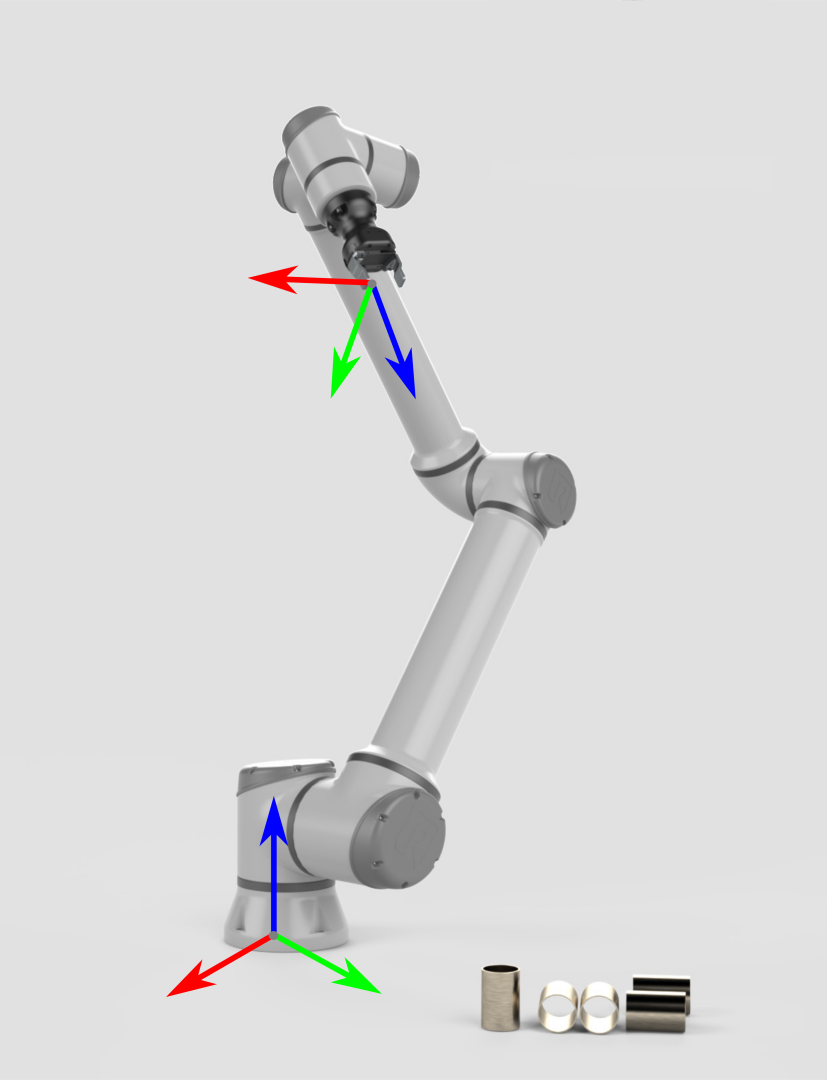To be able to pick an object, the robot controller needs to know the object’s pose (position and orientation) relative to the robot base frame. It also requires knowledge about the robot’s geometry. This combined information is sufficient to compute the joint angles that will move the end-effector/gripper towards the object.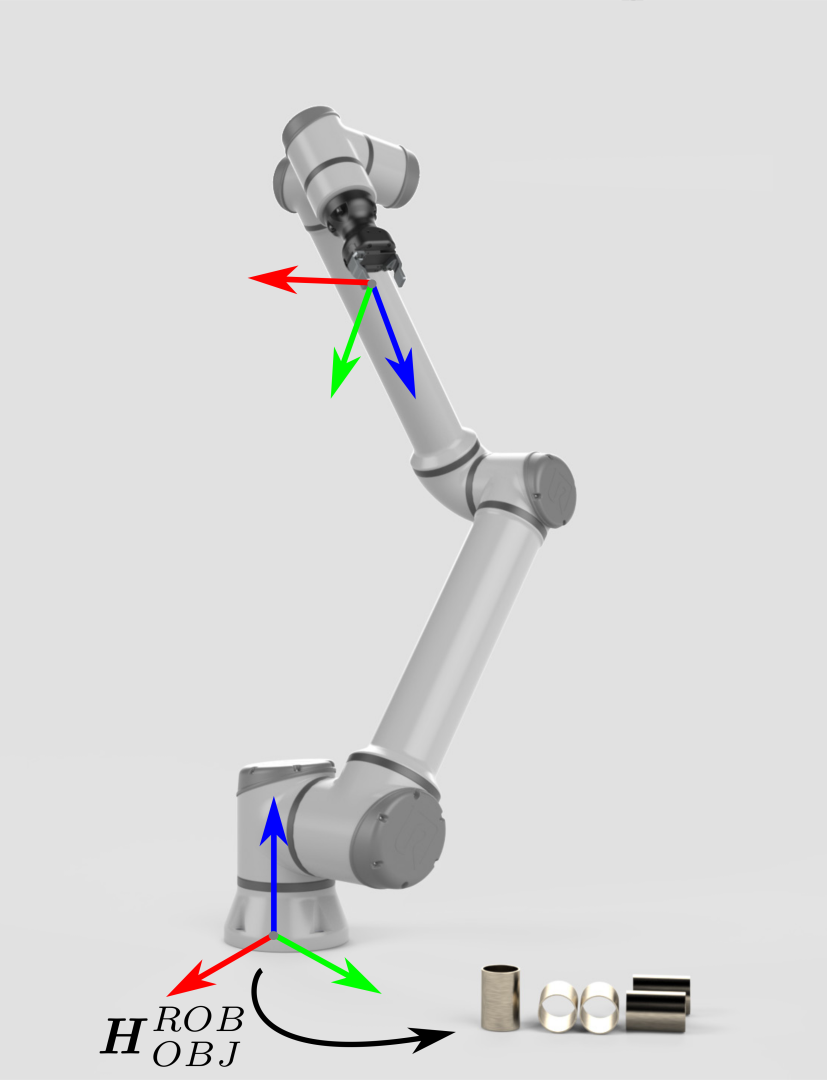Now, let’s assume that the pose of the object relative to the robot is unknown. That’s where Zivid 3D vision comes into play.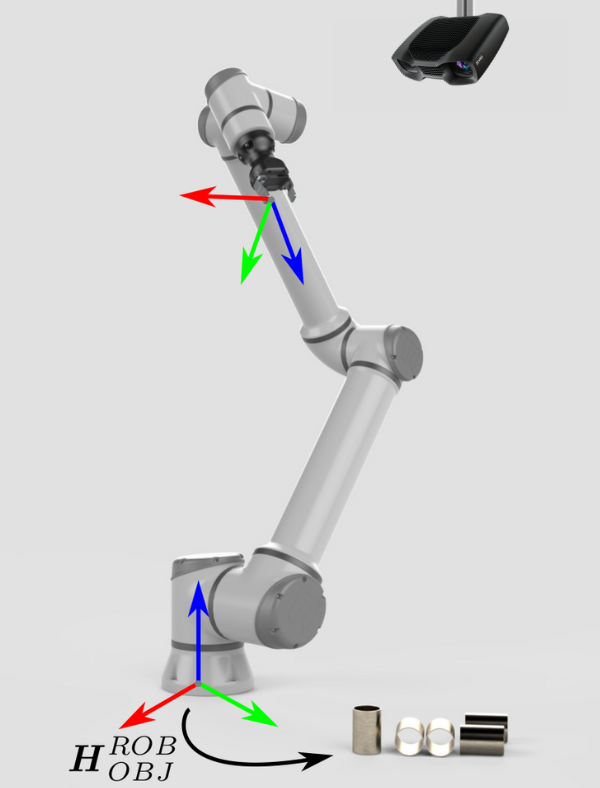Zivid point clouds are given relative to the Zivid camera’s coordinate system. The origin in this coordinate system is fixed at the middle of the Zivid imager lens (internal 2D camera). A machine vision software can run detection and localization algorithms on this collection of data points. It can determine the pose of the object in the Zivid camera’s coordinate system ($$H^{CAM}_{OBJ}$$).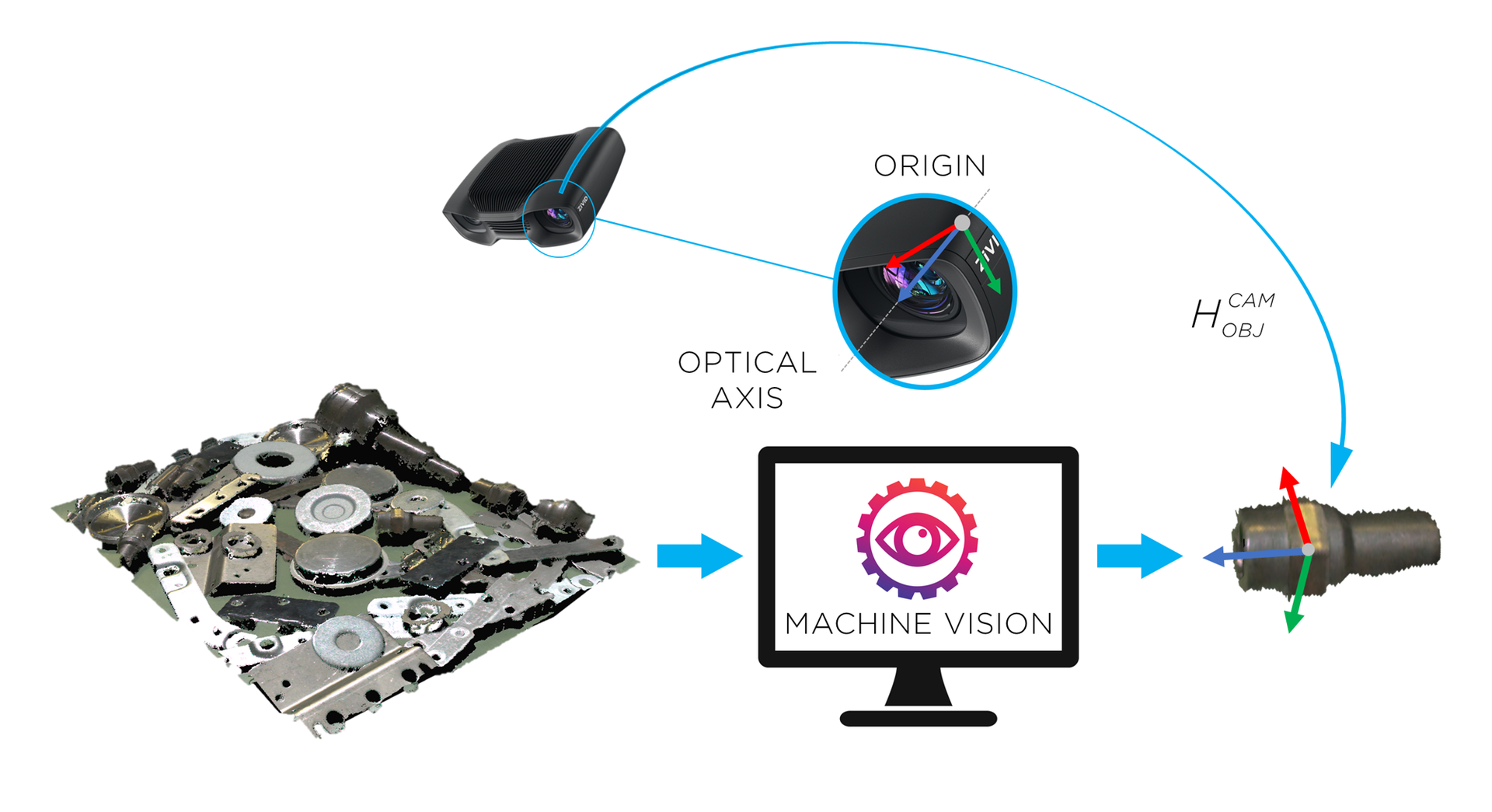Zivid camera can now see the object in its field of view, but relative to its own coordinate system. To enable the robot to pick the object it is necessary to transform the object’s coordinates from the camera coordinate system to the robot base coordinate system.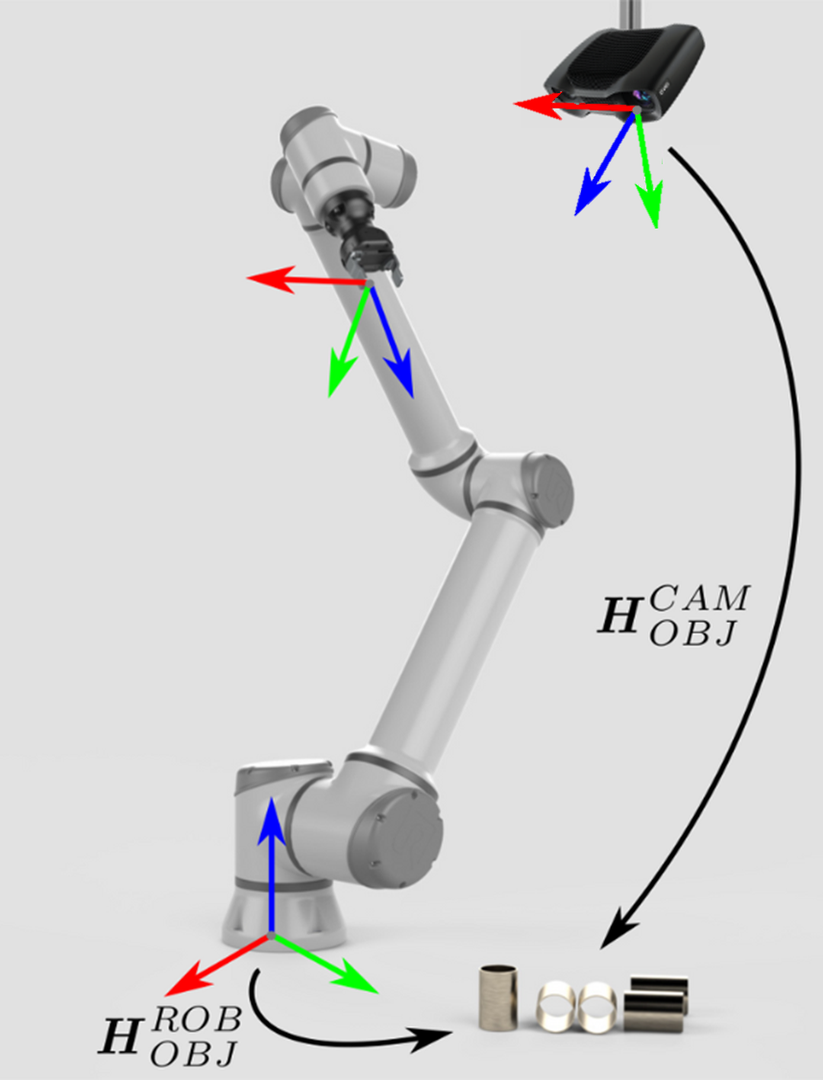The coordinate transformation that enables this is the result of hand-eye calibration. For eye-to-hand systems, it is the pose of the camera relative to the robot base ($$H^{ROB}_{CAM}$$) that is estimated with the hand-eye calibration. Once the pose circle is closed, it is possible to calculate one pose from the other poses in the circle. In this case, the pose of the object relative to the robot. This is found by post-multiplying the pose of the camera relative to the robot, with the pose of the object relative to the camera: $H^{ROB}_{OBJ}=H^{ROB}_{CAM} \cdot H^{CAM}_{OBJ}$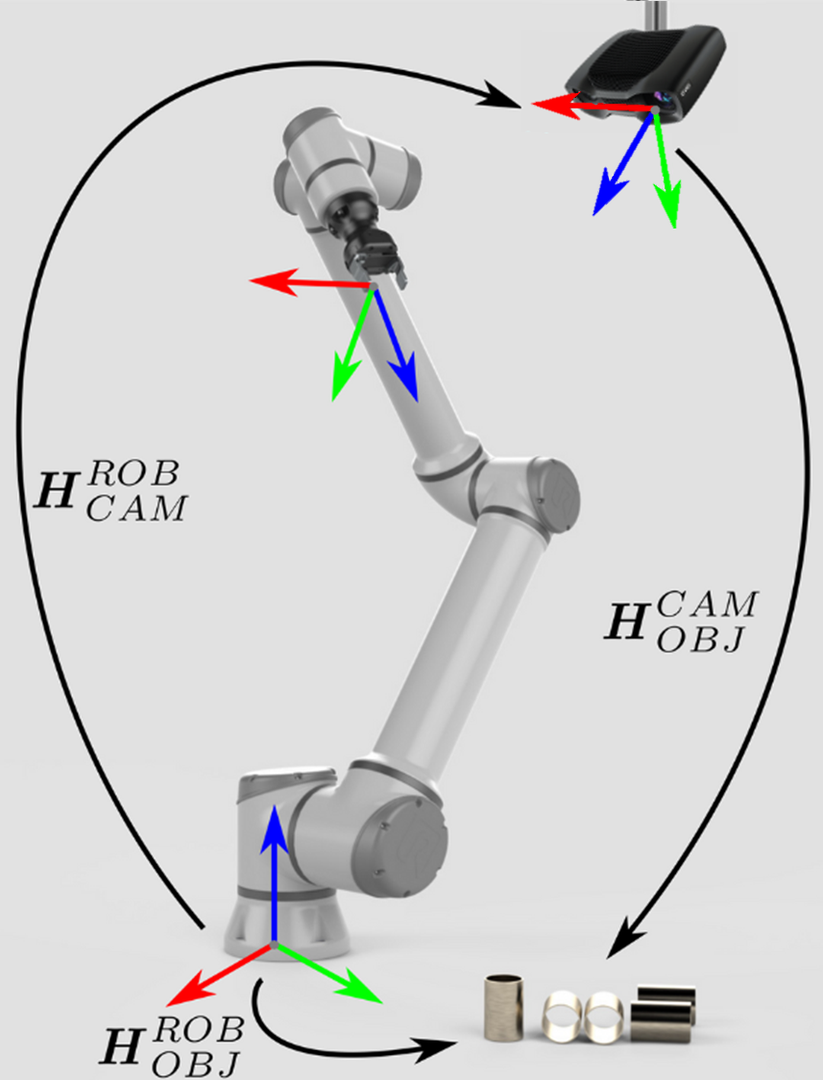## Eye-in-hand

How can a robot pick an object?

 The goal is the same for the eye-in-hand configuration. For a robot to pick an object, the object’s pose needs to be transformed from the camera’s coordinate system to that of the robot.In this case, the transformation is done indirectly: $H^{ROB}_{OBJ}=H^{ROB}_{EE} \cdot H^{EE}_{CAM} \cdot H^{CAM}_{OBJ}$ The pose of the end-effector relative to the base of the robot ($$H^{ROB}_{EE}$$) is known, and is provided by the robot controller. The pose of the camera relative to the end-effector ($$H^{EE}_{CAM}$$), which is in this case constant, is estimated from the hand-eye calibration.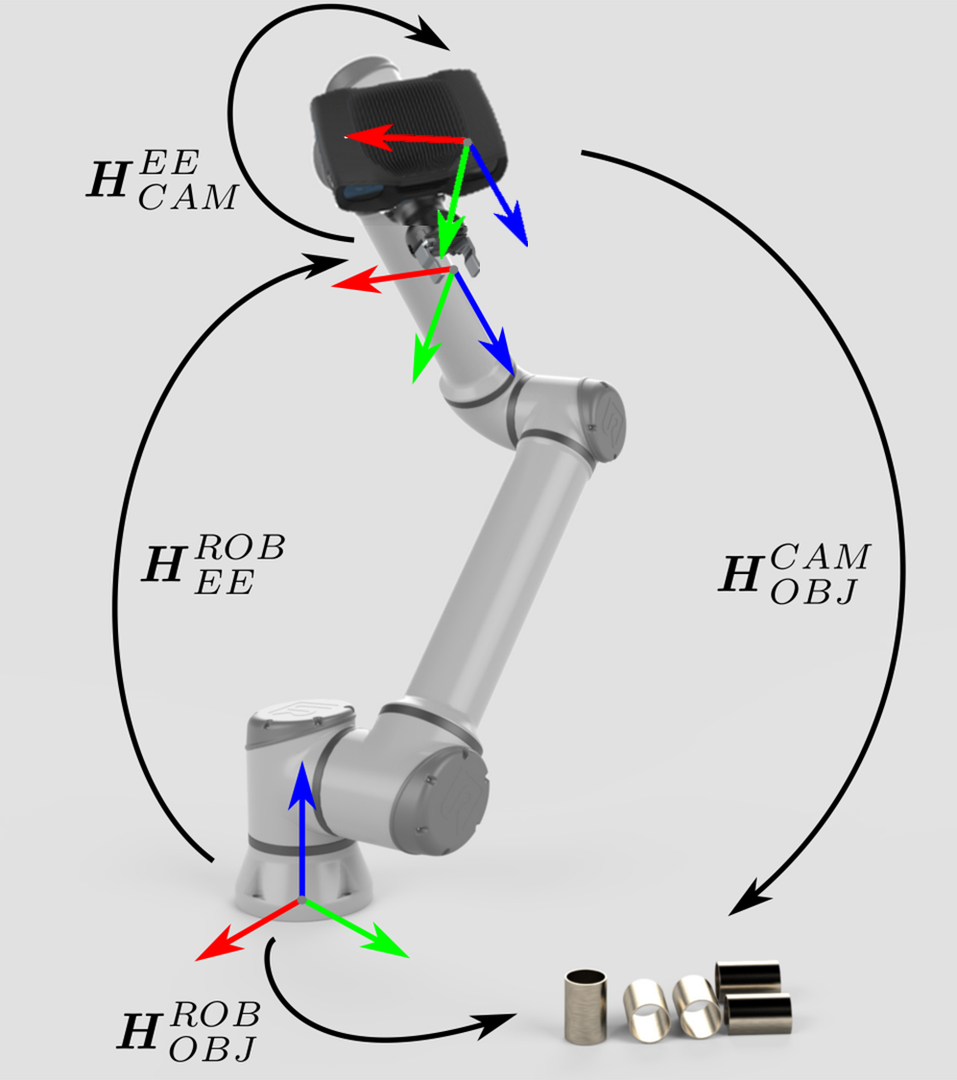Now that we’ve defined the hand-eye calibration problem, let’s see the Hand-Eye Calibration Solution.